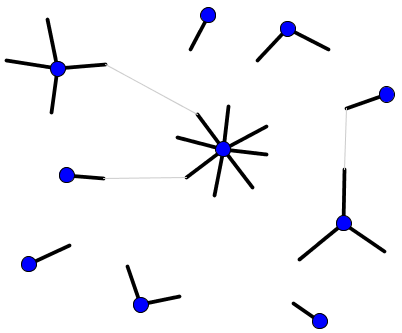# Math Insight

### Generating networks with a desired degree distribution

#### Motivation

The degree distribution is a handy tool for exploring properties of networks. Given a network or a probability distribution describing a random network model, it's a simple matter to calculate the degree distribution. One needs to either make a histogram of the degrees of all the network nodes or calculate the appropriate averages using a random network model.

Sometimes, though, we might want to go the other way around. For example, if we have a complex real world network and calculate its degree-distribution, we might be interested in exploring to what extent the degree distribution captures certain aspects of the network. If we could generate an ensemble of networks with the given degree distribution, we could compare the properties of the ensemble with the original network to see what properties are captured by the ensemble and what properties seem to depend on network structure other than the degree distribution.

Another reason to explore network models incorporating degree distributions is to develop random network models that add additional structure beyond the simplest random network models in which edges are generated independent, often called Erdös-Rényi random networks or ER random networks. Real world networks tend to have much broader degree distributions than in ER random networks, where all nodes have similar degrees. Hence, random network models that add minimal structure beyond a degree distribution can allow one to explore the consequences having a large spread of degrees, including hubs that have a much larger degree than most nodes.

#### The configuration model

The configuration model1,2 allows one to generate a network model that has exactly a prescribed degree distribution. It allows one to generate networks with the same degree distribution as a given network. One can simply cut all the edges in the network, so every node still retains its degree by the number of half-edges or stubs emanating from it. The result will be an even number of half-edges. To create new networks with the same degree, one simply needs to randomly pair all the half-edges, creating the new edges in the network.The configuration model generates every possible graph with the given degree distribution with equal probability.2 It naturally creates networks with multiple edges between nodes and self-connections between nodes. If such networks are unacceptable, one can reject those samples that have multiple or self-connections and try the algorithm again, repeating until one obtains a network without multiple or self-connections. However, in order to make sure one is uniformly sampling all the networks without multiple or self-connections that have the desired degree distribution, it is important than one starts generating the network from scratch when rejecting a network.3 If one simply deletes an offending edge and continues the algorithm, one alters the statistics of the resulting ensemble of networks.

#### An alternative model by Chung and Lu

One disadvantage of the configuration model is that it is not a logical extension of the ER random network model. If one gives the configuration model the (binomial) degree distribution of a ER random network model, the resulting ensemble of networks generated by this algorithm differs from the ER random network. For example, different samples from the same ER random network model will have slightly different degree distributions, as opposed to configuration model samples which all have exactly the same degree distribution.

One can extend the ER random network model to different degree distribution if one relaxes the requirement that all samples exactly reproduce the desired degree distribution. Instead, of assigning degrees to each node, one can one can simply assign each node's expected degree. Chung and Lu4,5 developed an algorithm to generate networks with these expected degrees. If one sets the expected degree of each node to be the same, one obtains the ER random network model from Chung and Lu's algorithm.

We described both above algorithms in terms of undirected graphs, but they can be simply extended to directed graphs. In this case, specifying the model is a little more complicated as one needs to specify the full two-dimensional degree distribution in terms of in- and out-degree.